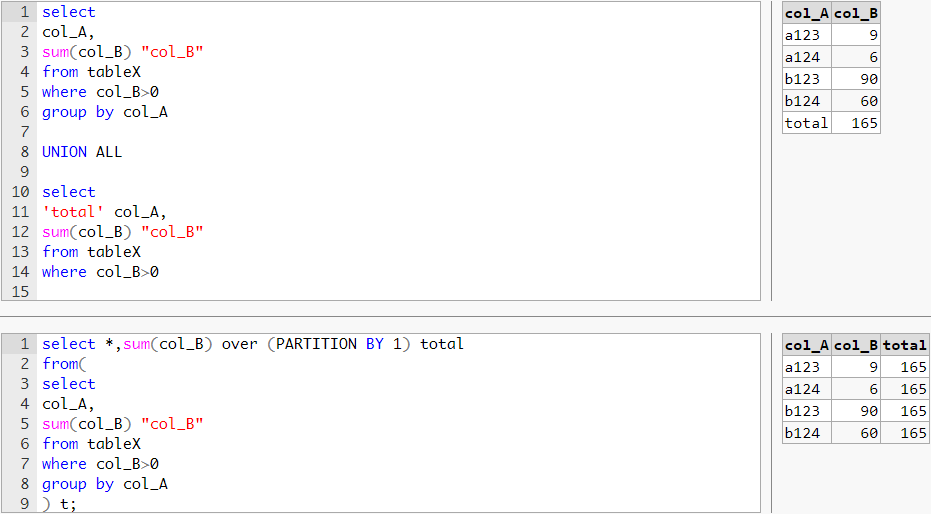#0

## SQL 問題 distinct 後加總後再加入總計

SQL語法如下，col_A跟col_B在同一個table，我想在下面結果再加一欄全部的col_B加總合計該怎麼寫？
select distinct(col_A) , sum(col_B)
from table
where col_B>0
group by col_A;

tlcric iT邦新手 5 級 ‧ 2019-03-14 09:45:30 檢舉

select substr(col_A,1,1) , sum(col_B) from tableX
where col_B > 0
and col_A <> ' '
group by substr(col_A,1,1)
/*撈欄位A(文字)取最左邊一碼，統計相同碼的加總
tableX
col_A col_B
A123 2
A124 5
B123 6
B124 8

A 7
B 14

tlcric iT邦新手 5 級 ‧ 2019-03-14 10:55:16 檢舉

### 4 個回答

1

``````select *,sum(col_B) over (PARTITION BY 1) total
from(
select
col_A,
sum(col_B) "col_B"
from table
where col_B>0
group by col_A
) t;
``````

2019/3/14補充：

``````select
col_A,
sum(col_B) "col_B"
from table
where col_B>0
group by col_A

UNION ALL

select
'total' col_A,
sum(col_B) "col_B"
from table
where col_B>0
``````tlcric iT邦新手 5 級 ‧ 2019-03-14 09:36:32 檢舉

tlcric iT邦新手 5 級 ‧ 2019-03-14 09:38:33 檢舉

1

``````select col_A, sum(col_B) as col_B
from tableX
where col_B>0
group by col_A
--
union all
select 'subtotal : ' + substring(col_A,1,1) as col_A, sum(col_B) as col_B
from tableX
where col_B>0
group by substring(col_A,1,1)
--
union all
select 'total' as col_A, sum(col_B) as col_B
from tableX
where col_B>0
``````
1
``````create table ithelp190314 (
id smallint generated always as identity
, cola text not null
, colb smallint not null
);

insert into ithelp190314 (cola, colb) values
('a', 1), ('a', 2), ('a', 3),('a', -1),
('b', 2), ('b', 4), ('b', 6),('b', -2);

--
select cola
, sum(colb)
from ithelp190314
where colb > 0
group by cola;

+------+-----+
| cola | sum |
+------+-----+
| a    |   6 |
| b    |  12 |
+------+-----+
(2 rows)

--
select cola
, sum(colb) as "sum(colb > 0)"
, NULL::bigint as "all sum(colb)"
, NULL::bigint as "all sum(abs(colb))"
from ithelp190314
where colb > 0
group by cola
union all
select '----', NULL, NULL, NULL
union all
select 'All '
, NULL
, sum(colb)
, sum(abs(colb))
from ithelp190314
;

+------+---------------+---------------+--------------------+
| cola | sum(colb > 0) | all sum(colb) | all sum(abs(colb)) |
+------+---------------+---------------+--------------------+
| a    |             6 |               |                    |
| b    |            12 |               |                    |
| ---- |               |               |                    |
| All  |               |            15 |                 21 |
+------+---------------+---------------+--------------------+
(4 rows)
````````````-- 另外一招
select cola
, sum(colb) as "sum(colb)"
, sum(abs(colb)) as "sum(abs(colb))"
from ithelp190314
group by rollup (cola)
order by cola NULLS LAST;

+------+-----------+----------------+
| cola | sum(colb) | sum(abs(colb)) |
+------+-----------+----------------+
| a    |         5 |              7 |
| b    |        10 |             14 |
|      |        15 |             21 |
+------+-----------+----------------+
(3 rows)
``````
1
``````select *
from ((
select col_A, sum(col_B)
from table
where col_B > 0
group by col_A
)union all(
select null, sum(col_B)
from table
where col_B > 0
)) as k
``````

``````select *
from ((
select substr(col_A,1,1) col_A
,sum(col_B) col_B
from tableX
where col_B > 0
and col_A <> ' '
group by substr(col_A,1,1)
)union all(
select null
,Sum(col_B)
from (
select substr(col_A,1,1) col_A
,sum(col_B) col_B
from tableX
where col_B > 0
and col_A <> ' '
group by substr(col_A,1,1)
) as k
)) as k
``````